CATEGORIES:

# Incidence matrices

Another common way to represent graphs is to use incidence matrices. Let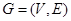be an undirected graph. Suppose thatare the vertices and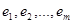are the edges of G. Then the incidence matrix with respect to this ordering of V and E is the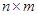matrix, where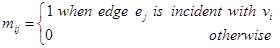.

Example. Represent the graph shown in Figure below with an incidence matrix.Solution: e1 e2 e3 e4 e5 e6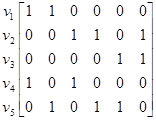Incidence matrices can also be used to represent multiple edges and loops. Multiple edges are represented in the incidence matrix using columns with identical entries, since these edges are incident with the same pair of vertices. Loops are represented using a column with exactly one entry equal to 1, corresponding to the vertex that is incident with this loop.

Example. Represent the pseudograph shown in Figure below using an incidence matrix.Solution: e1 e2 e3 e4 e5 e6 e7 e8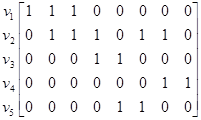Date: 2015-01-02; view: 1980

 <== previous page | next page ==> Representing graphs | Isomorphism of graphs
doclecture.net - lectures - 2014-2023 year. Copyright infringement or personal data (0.006 sec.)